Maths-
General
Easy

Question

# A truck covers a particular distance in 3 hours with the speed of 60 miles per hour. If the speed is increased by 30 miles per hour, find the time taken by the truck to cover the same distance.Hint:

## The correct answer is: 2 hours.

### Let the speed of the truck be represented as x and the time taken by the truck be represented as y. The time taken by the truck to cover a distance will decrease if the speed of the truck increases so we can conclude that x is in inverse relationship with y. Let’s say the proportional relationship is given asy =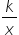……..(1)Step 1 of 2:It is given that the truck covers a particular distance in 3 hours with the speed of 60 miles per hour i.e. x = 60 and y = 3. Putting the values in equation (1)3 =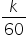k = 3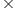60  = 180Step 2 of 2:Now we are asked to find the time taken by the truck to cover the same distance if the speed is increased by 30 miles per hour i.e the speed of the truck becomes 90 miles per hour. So, x = 90 and we need to find y. Putting the values in equation (1)y =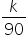Now, put the value of k = 180y =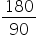y = 2 hoursFinal Answer: Hence, the time taken by the truck to cover the same distance if the speed is increased by 30 miles per hour is 2 hours.Note: This question can be solved by simply using the formula of speed( speed =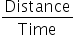).#### With Turito Foundation.#### Get an Expert Advice From Turito.